# alex dissolves 1. Alex dissolves 19.8 g solute into 38.0 g water and finds that crystals...

alex dissolves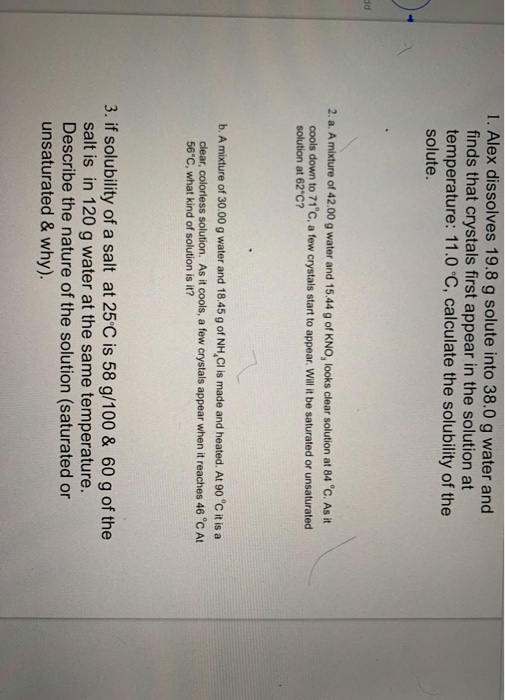1. Alex dissolves 19.8 g solute into 38.0 g water and finds that crystals first appear in the solution at temperature: 11.0 °C, calculate the solubility of the solute. 2. a. A mixture of 42.00 g water and 15.44 g of KNO, looks clear solution at 84 °C. As it cools down to 71°C, a few crystals start to appear. Will it be saturated or unsaturated solution at 62"C? b. A mixture of 30.00 g water and 18.45 g of NH,Cl is made and heated. At 90 °C it is a clear, colorless solution. As it cools, a few crystals appear when it reaches 46 °C At 56°C, what kind of solution is it? 3. if solubility of a salt at 25°C is 58 g/100 & 60 g of the salt is in 120 g water at the same temperature. Describe the nature of the solution (saturated or unsaturated & why).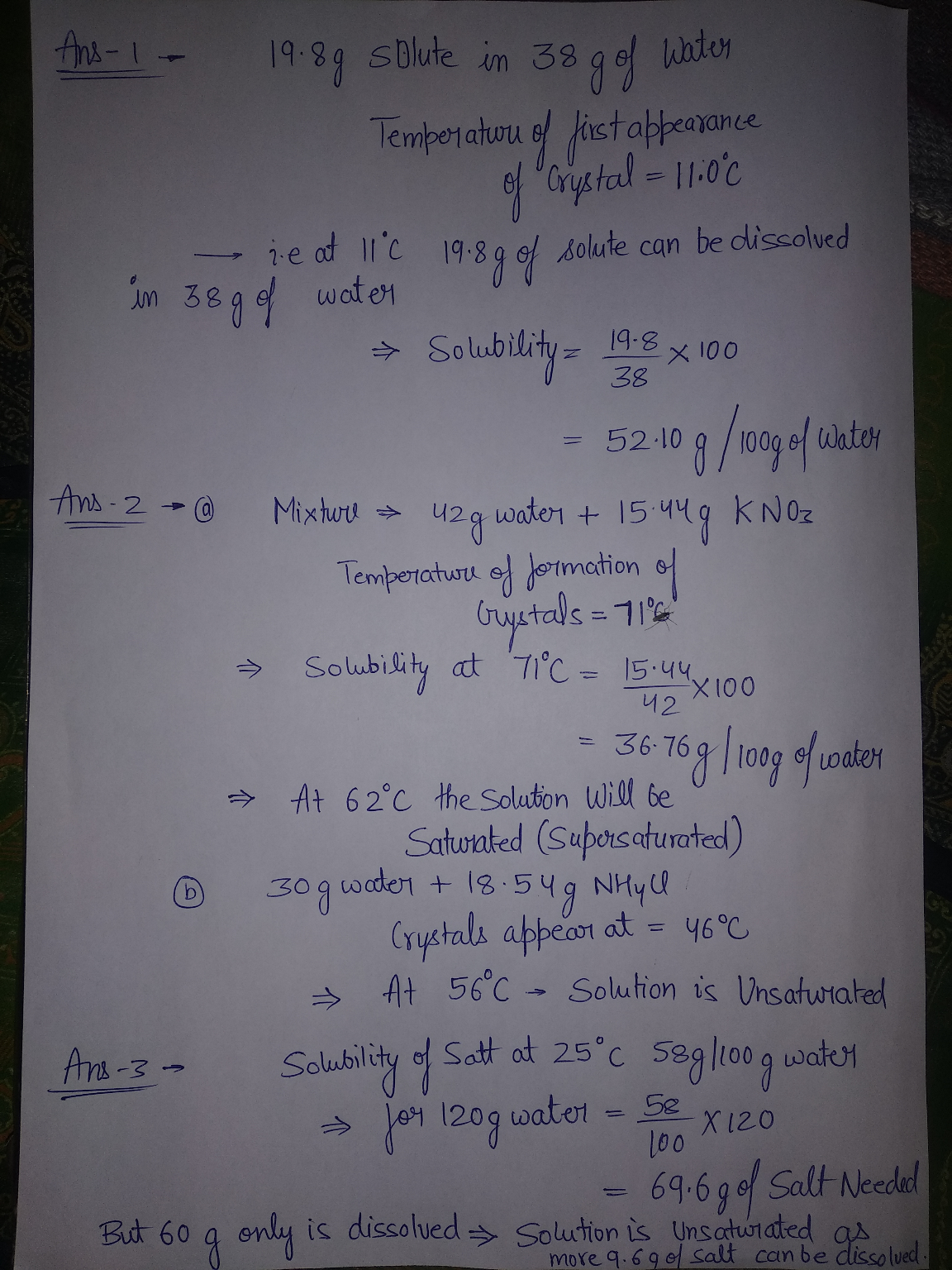#### Earn Coin

Coins can be redeemed for fabulous gifts.

Similar Homework Help Questions
• ### is it saturated, unsaturated, or supersaturated A mixture of 30.00 g water and 18.45 g of...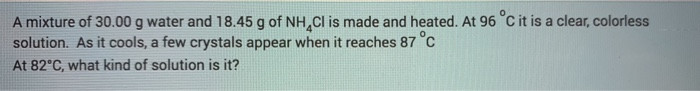is it saturated, unsaturated, or supersaturated A mixture of 30.00 g water and 18.45 g of NH Cl is made and heated. At 96 °C it is a clear, colorless solution. As it cools, a few crystals appear when it reaches 87°C At 82°C, what kind of solution is it?

• ### How would you describe a KCIOg solution at 80°C if it contains 30 g of solute...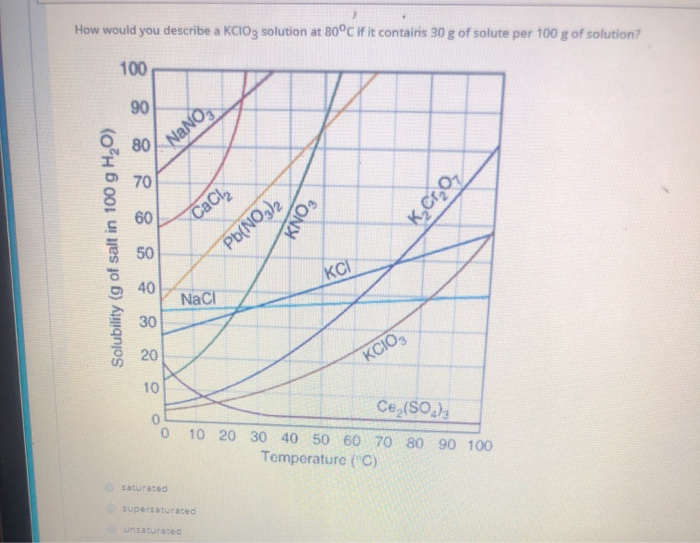How would you describe a KCIOg solution at 80°C if it contains 30 g of solute per 100 g of solution? 80/NANO K,C1,02 Cache KNO, Solubility (g of salt in 100 g H2O) Pb(NO3)2 NaCl KCIO, Ce (80.), 0 10 20 30 40 50 60 70 80 90 100 Temperature (°C) saturated Supersaturated Unsaturated

• ### G of sodium nitrate (NaNO,) dissolves in 100 g of water at 20°C. Is this solution saturated or un...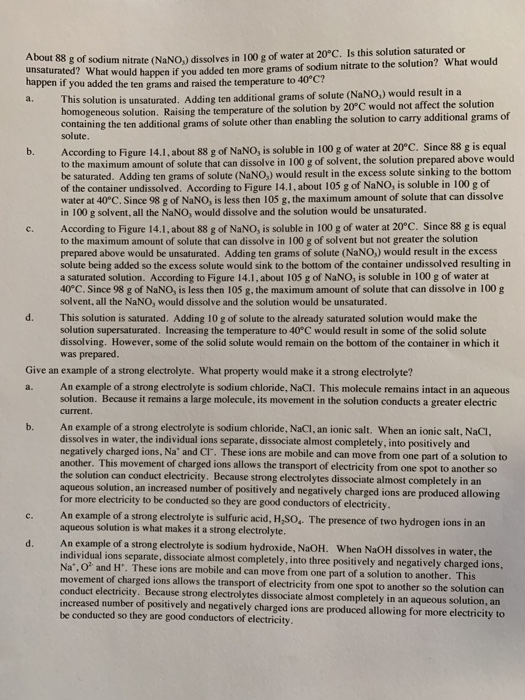g of sodium nitrate (NaNO,) dissolves in 100 g of water at 20°C. Is this solution saturated or unsaturated? Wha t would happen if you added ten more grams of sodium nitrate to the solution? What would if you added the ten grams and raised the temperature to 40 C? lution is unsaturated. Adding ten additional grams of solute (NaNO,) would result in a homo geneous solution. Raising the temperature of the solution by 20°C would not affect the solution...

• ### Saturated, Unsaturated & Supersaturated Solutions Solution, in which the concentration of solute is that determined by...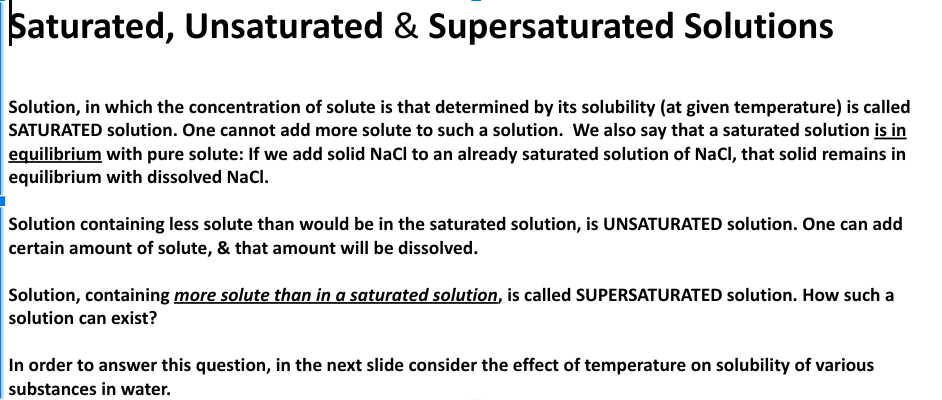Saturated, Unsaturated & Supersaturated Solutions Solution, in which the concentration of solute is that determined by its solubility (at given temperature) is called SATURATED solution. One cannot add more solute to such a solution. We also say that a saturated solution is in equilibrium with pure solute: If we add solid Nacl to an already saturated solution of NaCl, that solid remains in equilibrium with dissolved Naci. Solution containing less solute than would be in the saturated solution, is UNSATURATED...

• ### Classify each of the following solutions as an unsaturated, a saturated, or supersaturated solution. a solution...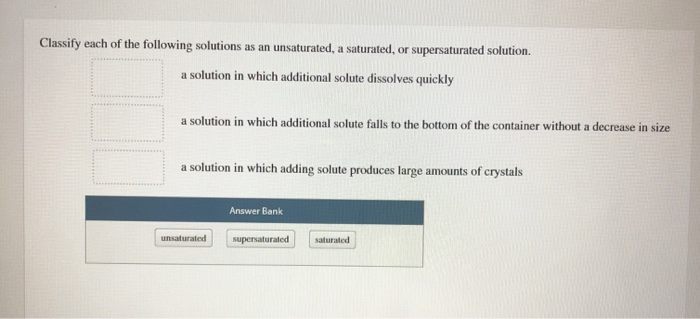Classify each of the following solutions as an unsaturated, a saturated, or supersaturated solution. a solution in which additional solute dissolves quickly a solution in which additional solute falls to the bottom of the container without a decrease in size a solution in which adding solute produces large amounts of crystals Answer Bank unsaturated supersaturated saturated Arrange these compounds by their expected solubility in hexane, CH- Most soluble in hexane Least soluble in hexane Answer Bank 4,0 CHg C,HOH The...

• ### Solute KMnO4 Soluble/Not Soluble in Water (A.1) Cyclohexane (A.2) Identify the Solute as Polar or Nonpolar...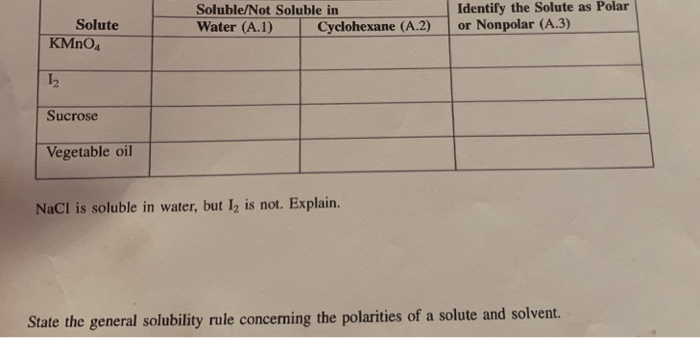Solute KMnO4 Soluble/Not Soluble in Water (A.1) Cyclohexane (A.2) Identify the Solute as Polar or Nonpolar (A.3) Sucrose Vegetable oil NaCl is soluble in water, but I, is not. Explain. State the general solubility rule concerning the polarities of a solute and solvent. Calculations B.4 Solubility is expressed as the number of grams of solute in 100 mL of water. Because you used a sample of 5.0 mL of water, the mass of the solute you measured out and the...

• ### A student is evaluating the solubility potassium (KClO3) at various temperatures. She dissolves 2.50 g of...

A student is evaluating the solubility potassium (KClO3) at various temperatures. She dissolves 2.50 g of KCLO3 in a total volume of 7.5 mL of water. a. Calculate the concentration of KClO3 in this solution. b. The student heats the solution up to 80 degrees Celsius, the removes the test tube containing the salt solution from the hot water bath. At what temperature would you expect to see the formation of salt crystals in the solution? c. If the solution...

• ### Principles of Inorganic Chemistry! Week 5 Effect of Temperature on Solubility of a Salt In this experiment, you w...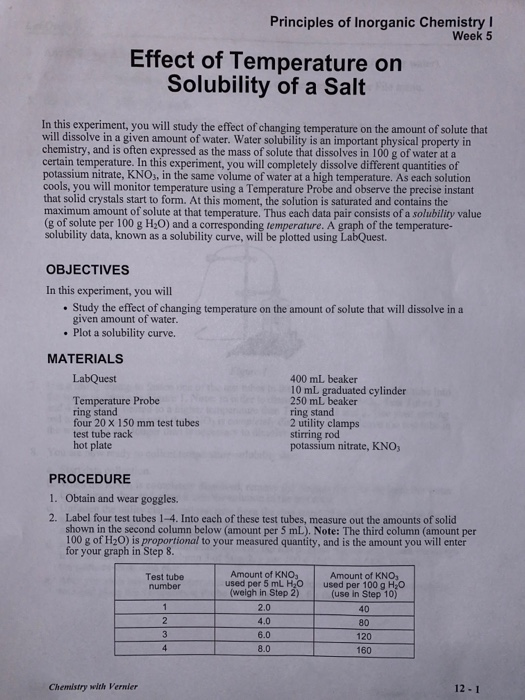Principles of Inorganic Chemistry! Week 5 Effect of Temperature on Solubility of a Salt In this experiment, you will study the effect of changing temperature on the amount of solute that will dissolve in a given amount of water. Water solubility is an important physical property in chemistry, and is often expressed as the mass of solute that dissolves in 100 g of water at a certain temperature. In this experiment, you will completely dissolve different quantities of potassium nitrate,...

• ### E3. Answer the following questions from the given solubility curve. Table G Solubility Curves KI NANO,...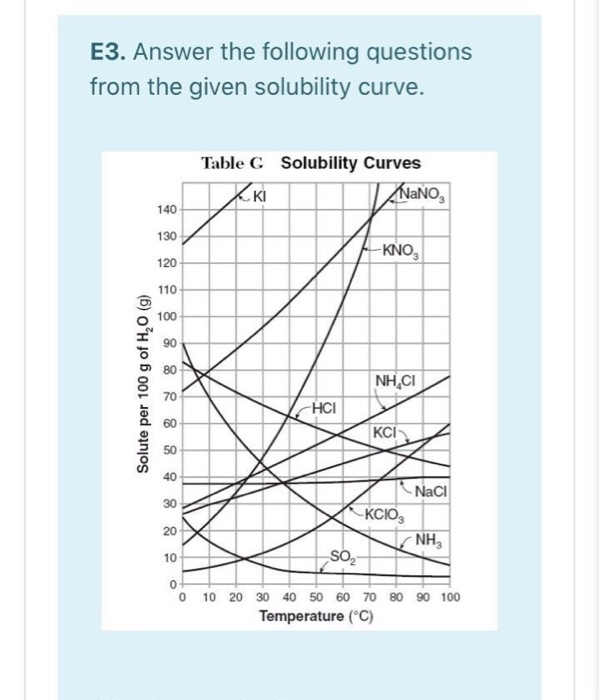E3. Answer the following questions from the given solubility curve. Table G Solubility Curves KI NANO, 140 130 -KNO, 120 110 100 90 80 Solute per 100 g of H, (g) NHẠCI 70 HCI 60 KCI 50 40 30 Naci -KCIO, NH 20 10 SO2 0 0 10 20 30 40 50 60 70 80 90 100 Temperature (°C) (a) How much of the potassium chloride will dissolve and how much remain undissolved at the bottom of the beaker, when...

• ### ​At 20°C, the solubility of KCl is 34 g/100 g water. In the laboratory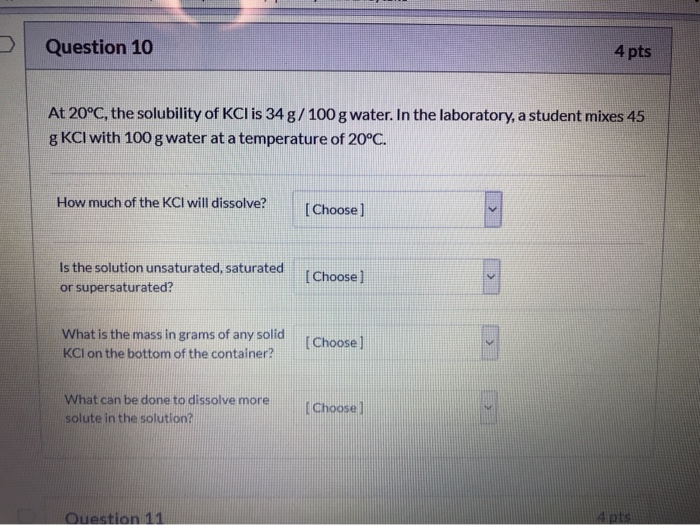At 20°C, the solubility of KCl is 34 g/100 g water. In the laboratory, a student mixes 45 g KCl with 100 g water at a temperature of 20°C. How much of the KCl will dissolve?Is the solution unsaturated, saturated or supersaturated? What is the mass in grams of any solid KCl on the bottom of the container? What can be done to dissolve more solute in the solution?

Free Homework Help App